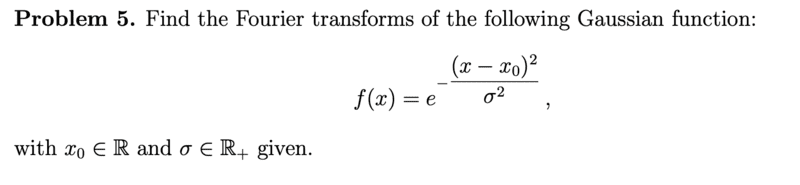# Compute the integral of the Gaussian

docnet
Homework Statement:
.
Relevant Equations:
.why does it say transforms? is there more than one Fourier transform?? we learned in class that the inverse Fourier transform of the Fourier transform of ##f## is ##f##, so there should be just one right? I'm uncertain of how to calulate this integral though.. Mr Wolfram showed me an indefinite integral involving an error function, but there has to be a different way to integrate it because we didn't learn about the error function. Mr Wolfram says

$$\int_{-\infty}^\infty fe^{-ikx}dx=\sqrt{\pi}\sigma e^{(\frac{\sigma k}{2})^2}e^{-ikx_0}$$

how does one go from the beginning to the finish?

ergospherical
Write\begin{align*}
\tilde{f}(k) &= \int_{-\infty}^{\infty} \mathrm{exp}\left( -ikx - \frac{(x-x_0)^2}{\sigma^2} \right) dx \\
&= e^{-ikx_0} \int_{-\infty}^{\infty} \mathrm{exp}\left( -ik(x-x_0) - \frac{(x-x_0)^2}{\sigma^2} \right) dx\end{align*}from here a 'complete-the-square' substitution like ##u = \dfrac{x-x_0}{\sigma} + \dfrac{i\sigma k}{2}## looks helpful, but take some care to justify the limits of the integral over ##u## given that ##u## is complex.

Last edited:
•docnet
docnet
Write\begin{align*}
\tilde{f}(k) &= \int_{-\infty}^{\infty} \mathrm{exp}\left( -ikx - \frac{(x-x_0)^2}{\sigma^2} \right) dx \\
&= e^{-ikx_0} \int_{-\infty}^{\infty} \mathrm{exp}\left( -ik(x-x_0) - \frac{(x-x_0)^2}{\sigma^2} \right) dx\end{align*}from here a 'complete-the-square' substitution like ##u = \dfrac{x-x_0}{\sigma} + \dfrac{i\sigma k}{2}## looks helpful, but take some care to justify the limits of the integral over ##u## given that ##u## is complex.
\begin{align} \tilde{f}(k) =& \int_{-\infty}^{\infty} \mathrm{exp}\left( -ikx - \frac{(x-x_0)^2}{\sigma^2} \right) dx \\
=e^{-ikx_0} &\int_{-\infty}^{\infty} \mathrm{exp}\left( -ik(x-x_0) - \frac{(x-x_0)^2}{\sigma^2} \right)dx\\
=e^{-ikx_0} &\int_{-\infty}^{\infty} \mathrm{exp}\left( -\frac{\sigma^2i^2k^2}{4}+\frac{\sigma^2i^2k^2}{4}-ik(x-x_0) - \frac{(x-x_0)^2}{\sigma^2} \right)dx\\
=e^{-ikx_0-\frac{\sigma^2i^2k^2}{4}} &\int_{-\infty}^{\infty} \mathrm{exp}\left( -(\frac{\sigma i k}{2}+\frac{x-x_0}{\sigma})^2 \right)dx\end{align}
let ##u = \dfrac{x-x_0}{\sigma} + \dfrac{i\sigma k}{2}##. since ##u## is complex, it leads to integrating for ##u\in\{(-\infty,\infty)\times \{0\}\}##.
\begin{align}&\sigma e^{-ikx_0-\frac{\sigma^2i^2k^2}{4}}\int_ u e^{-u^2}du\\
=&\sigma e^{-ikx_0-\frac{\sigma^2i^2k^2}{4}} \Big[\frac{\sqrt{\pi}}{2}\text{erf}(u)\Big]_u\\
=&\sigma \sqrt{\pi} e^{-ikx_0+\frac{\sigma^2k^2}{4}}\end{align}

Last edited:
docnet
is it ok?
:)